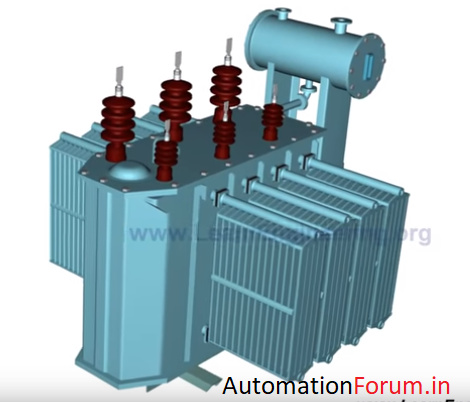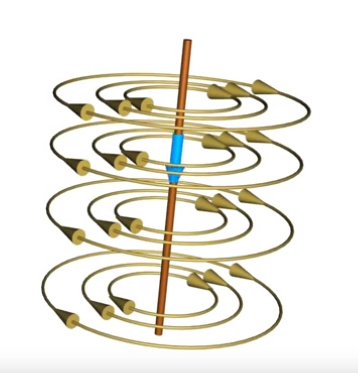# How does a transformer work?

Transformers are capable of receiving AC power at one voltage and delivering at another voltage. In this way, they help to achieve better transformation efficiency while transferring the power over longer distances.Let us consider the working of a three-phase transformer

## Working principle

The basic working principle of a transformer is based on the principle of electromagnetic induction. According to this a varying magnetic flux associated with a loop will induce an electromotive force across them. Such a fluctuating magnetic field easily be produced by a coil and an alternating emf system.

A current carrying conductor produces a magnetic field around it. The magnetic field produced by the coils is as shown in the figure.With the fluctuating nature of the alternating current, the magnetic field associated with the coil will also fluctuate. This magnetic flux will link to the secondary winding with the help of a core made up of ferromagnetic material. This fluctuating magnetic field will induce a magnetic emf in the secondary coil due to electromagnetic induction.

Since the turns are arranged in a series as shown in the above figure, the net emf induced across the winding will be the sum of individual EMFs induced in each turn.

Es= es * Ns

N is the number of turns

E is the emf per turn

Since the same magnetic field is passing through the primary and the secondary coils, the emf per turn for primary and secondary coils, the emf per turn for the primary and the secondary coil will be the same.

Therefore, ep=es

The emf per turn for the primary coil is related to the applied input voltage. As a result, the induced emf in the secondary coil is

es=(Ep/Np) * Ns

This means that if fewer turns are there in the secondary coil than in the primary coil, then one can lower the voltage as shown in the figure below.

Es=(Ep/Np) * Ns

Ns < Np

Es < Ep

For the reversed case, one can increase the voltage.

Es=(Ep/Np) * Ns

Ns > Np

Es > Ep

But since energy is conserved, the primary and the secondary currents have to obey the following relationship.

Es Is = Ep Ip

Three-phase transformers used such three single-phase transformers as shown in above figure but with slightly different coil configuration. Here the primary and the secondary coils are placed concentrically. Likewise, three such windings are employed in three-phase transformers.